Chemistry - 2013 Past Year Paper

# Chemistry - 2013 Past Year Paper - IIT JAM

Test Description

## 30 Questions MCQ Test IIT JAM Past Year Papers and Model Test Paper (All Branches) - Chemistry - 2013 Past Year Paper

Chemistry - 2013 Past Year Paper for IIT JAM 2023 is part of IIT JAM Past Year Papers and Model Test Paper (All Branches) preparation. The Chemistry - 2013 Past Year Paper questions and answers have been prepared according to the IIT JAM exam syllabus.The Chemistry - 2013 Past Year Paper MCQs are made for IIT JAM 2023 Exam. Find important definitions, questions, notes, meanings, examples, exercises, MCQs and online tests for Chemistry - 2013 Past Year Paper below.
Solutions of Chemistry - 2013 Past Year Paper questions in English are available as part of our IIT JAM Past Year Papers and Model Test Paper (All Branches) for IIT JAM & Chemistry - 2013 Past Year Paper solutions in Hindi for IIT JAM Past Year Papers and Model Test Paper (All Branches) course. Download more important topics, notes, lectures and mock test series for IIT JAM Exam by signing up for free. Attempt Chemistry - 2013 Past Year Paper | 30 questions in 180 minutes | Mock test for IIT JAM preparation | Free important questions MCQ to study IIT JAM Past Year Papers and Model Test Paper (All Branches) for IIT JAM Exam | Download free PDF with solutions
 1 Crore+ students have signed up on EduRev. Have you?
Chemistry - 2013 Past Year Paper - Question 1

### The most polar compound among the following is:

Chemistry - 2013 Past Year Paper - Question 2

### Which one of the following order of the carbonates is Correct for their decomposition temperature?

Detailed Solution for Chemistry - 2013 Past Year Paper - Question 2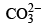is a polyatomic anion In case of polyatomic anion, Thermal stability of decomposition temperature is governed by covalent character

Thermal stability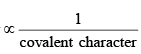Be 2+ Mg2+ Ca2+ Sr2+ Ba2+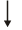Least size of cationMaximum covalent characterLeast thermally stable

∴BeCO3 < MgCO3 < CaCO3 < SrCO3 < BaCO3 Correct option is (b)

Chemistry - 2013 Past Year Paper - Question 3

### The Correct order of CO vibrational stretching frequency in the following complexes is (I) (PF3)3Mo(CO)3 (II) (PCl3)3Mo(CO)3 (III) {P(OMe)3}3Mo(CO)3

Detailed Solution for Chemistry - 2013 Past Year Paper - Question 3

Since the π-accepters attached to central metal cause the increase in the B.O. of CO, CO vibrational frequency increases. The order of π-acceptor is PF3 > PCl3 > P(OR)3 Therefore, order of vibrational stretching frequency is {P(OMe3)3} MO(CO)3 < (PCl3)3MO(CO)3 < (PF3)3MO(CO)3.
Correct option is (b)

Chemistry - 2013 Past Year Paper - Question 4

Among the following, the ligand that BEST stabilizes low oxidation state of tungsten (W) is

Detailed Solution for Chemistry - 2013 Past Year Paper - Question 4

Low oxidation state of W is stabilized by CO because of M →CO back p-bonding.
Correct option is (b)

Chemistry - 2013 Past Year Paper - Question 5

The function y = x exp (–x2) has a minimum at x =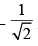The second derivative of the function at
the minimum is

Detailed Solution for Chemistry - 2013 Past Year Paper - Question 5

y =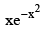minimum at x =second derivative of the function.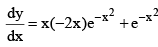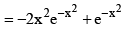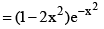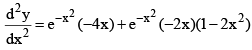Put x =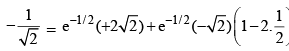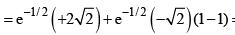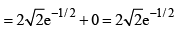Chemistry - 2013 Past Year Paper - Question 6

For a particular reaction at constant temperature, a plot of inverse of reactant concentration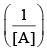versus time is a straight line with a slope of 4.0 × 10–2 L mol–1 s–1. The time required (in seconds) for 1.0 M of reactant to decrease to 0.25 M is:

Detailed Solution for Chemistry - 2013 Past Year Paper - Question 6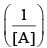vs ‘t’ graph gives straight line for a second order reaction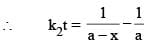... (1)

Or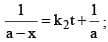y = mx + C

Slope = k2 = 4 × 10–2 L mol–1 sec–1

And a = 1.0 M      and a – x = 0.25 M

Therefore, (1), 4 × 10–2  t =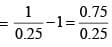⇒ t = 75

Correct option is (c)

Chemistry - 2013 Past Year Paper - Question 7

For a physisorption process, which one of the following statements is NOT correct?

Detailed Solution for Chemistry - 2013 Past Year Paper - Question 7

Physisorption is a reversible process in which the adsorbate molecules are attached to the adsorbent surface by weak vander waals forces preferably at low temperature.
Correct option is (c)

Chemistry - 2013 Past Year Paper - Question 8

The product of the following reaction is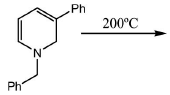Detailed Solution for Chemistry - 2013 Past Year Paper - Question 8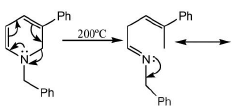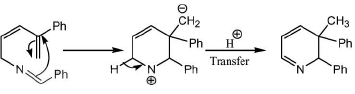Chemistry - 2013 Past Year Paper - Question 9

The Correct order of stability of the following carbonium ions is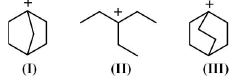Detailed Solution for Chemistry - 2013 Past Year Paper - Question 9

Carbocation at bridge head position is less stable because their rigid framework.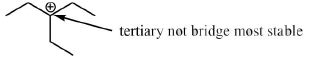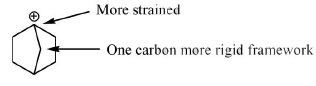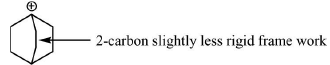So, stability of the carbocation follows the order.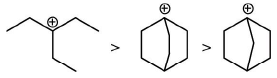Correct option is (d)

Chemistry - 2013 Past Year Paper - Question 10

Which one of the following statements is Correct?

Detailed Solution for Chemistry - 2013 Past Year Paper - Question 10

Because nucleic acids are polymer whose monomer is nucleotides not protein. So, (b) not correct.
Proteins are generally acts as enzyme but not vitamins. Proteins do not contain genetic information, its DNA which contain genetic information.
Correct option is (a)

*Answer can only contain numeric values
Chemistry - 2013 Past Year Paper - Question 11

The reaction of anhydrous FeCl2 with sodium-pentadienyl in ether gives an air-stable diamagnetic orange solid, which on oxidation gives an air-sensitive paramgnetic blue-green compound in solution.
The blue-green compound is _____________

Detailed Solution for Chemistry - 2013 Past Year Paper - Question 11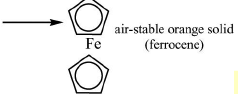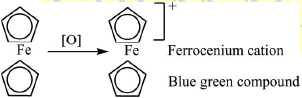*Answer can only contain numeric values
Chemistry - 2013 Past Year Paper - Question 12

CaO, VO and MnO have octahedral coordination of the metal ions in a rock-salt structure. The correct increasing order of their lattice enthalpies is

Detailed Solution for Chemistry - 2013 Past Year Paper - Question 12

CaO < MnO < VO

Because lattice energy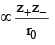Chemistry - 2013 Past Year Paper - Question 13

The structure of sulphur dioxide molecule (SO2) may be given as:

*Answer can only contain numeric values
Chemistry - 2013 Past Year Paper - Question 14

The vapour pressures of solid and liquid chlorine are given by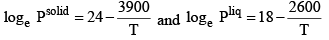where Psolid and Pliq are the vapour pressures (in Torr) of solid and liquid chlorine near the triple point, respectively and T is the absolute temperature. The ratio of the slope of the solid-gas curve to the slope of the liquid gas curve at the triple point in the P-T diagram is________

Detailed Solution for Chemistry - 2013 Past Year Paper - Question 14

Log P(solid ) =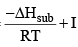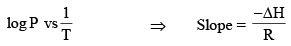Now,

Slope of sublimation curve = – 3900 Slope of vapour pressure curve = –2600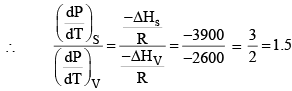*Answer can only contain numeric values
Chemistry - 2013 Past Year Paper - Question 15

For unnormalized wave-function ,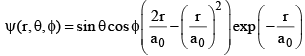the number of radial node(s) is __________

Detailed Solution for Chemistry - 2013 Past Year Paper - Question 15

Un normalized wave function ψ(r, θ, φ) =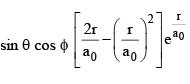Radial node is n – ℓ – 1 for given function n = 3, l = 1 Because one node at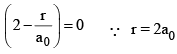Function written as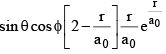Function is He+ because the part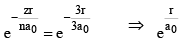Function related to 3px orbitals.

*Answer can only contain numeric values
Chemistry - 2013 Past Year Paper - Question 16

A hypothetical element (atomic weight = 300) crystallizes in a simple cubic lattice. For this crystal, the first order X-ray diffraction with wavelength of 5 Å appears at an angle of 30º. The density of the crystal is _____________g cm–3. [Avogadro number, NA = 6.02 × 1023]

Detailed Solution for Chemistry - 2013 Past Year Paper - Question 16

According to Bragg’s equation 2d sinθ = nλ ⇒ 2d sin 30 = 1 × 5 Å ⇒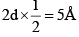⇒ d = 5Å
And for SCC, d = a = 5Å = 5 × 108 cm
Now density will be given as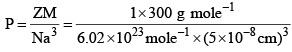= 3.9867 g cm–3

*Answer can only contain numeric values
Chemistry - 2013 Past Year Paper - Question 17

MnO4 (aq) + Zn(s) + H3O+ (aq) → Mn2+ (aq) + Zn2+ (aq) + H2O (ℓ) For the above reaction if the equilibrium constant at 298 K is represented by 10x, then the value of X is _________

[Given: The standard cell potential Eº = 2.4 V and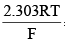= 0.06 V at 298 K]

Detailed Solution for Chemistry - 2013 Past Year Paper - Question 17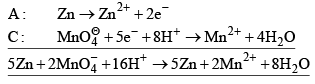n = 10
So,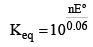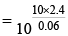x = 400

*Answer can only contain numeric values
Chemistry - 2013 Past Year Paper - Question 18

The rotational energy barrier between the most stable and the least stable conformations of 2, 3-dimethylbutane along C2–C3 bond is ___________kcal mol–1. [Given: The energies (kcal mol–1) for H/CH3 eclipsing = 1.8, CH3/CH3 eclipsing = 2.9 and CH3/ CH3 gauche = 0.9]

Detailed Solution for Chemistry - 2013 Past Year Paper - Question 18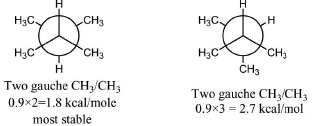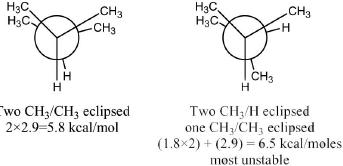Rotational energy barrier = 6.5 × 1.8 = 4.7 kcal/mol

*Answer can only contain numeric values
Chemistry - 2013 Past Year Paper - Question 19

The number of peaks or signals in 1H NMR of N, N-dimethylformamide (DMF) at 25ºC is ______

Detailed Solution for Chemistry - 2013 Past Year Paper - Question 19

Distinguishable hydrogen are indicated by a and b here. at 25ºC two signals.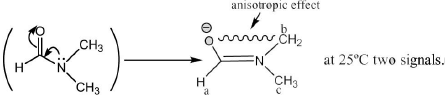*Answer can only contain numeric values
Chemistry - 2013 Past Year Paper - Question 20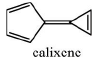Calixene is a polar hydrocarbon with a high dipole moment. The most stable dipolar canonical structure is _________

Detailed Solution for Chemistry - 2013 Past Year Paper - Question 20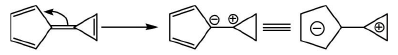Canonical

Because in this form both the rings are aromatic hence stable.

Chemistry - 2013 Past Year Paper - Question 21

A mixture of C3H8 and oxygen in 1L closed vessel has an internal pressure of 4 atm at 100ºC. When the mixture is ignited, the reaction produces CO2(g) and H2O(g) until all oxygen is consumed.
After the reaction, pressure of the vessel is 4.2 atm at the same temperature. Calculate the weight of oxygen present before the reaction. [Gas constant, R = 0.082 L atm mol–1 K–1].

Detailed Solution for Chemistry - 2013 Past Year Paper - Question 21

C3H8(g) + 5O2(g) → 3CO2(g) + 4H2O(g)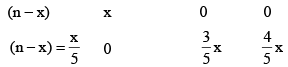Initial number of moles, n =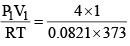= 0.1306

Final number of moles, nf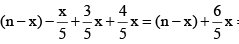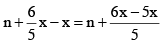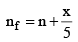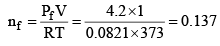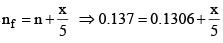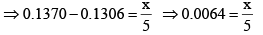x = 5 × 0.0064 ⇒ x = 0.032
weight of O2 = x × 32 = 0.032 × 32 = 1.024 gm

Chemistry - 2013 Past Year Paper - Question 22

The following reaction is carried out at 1 atm and 300 K 2H2(g) + O2(g) → 2H2O (l) ΔU

for the above reaction is 550 kJ. Assuming ideal gas behaviour for H2 and O2, calculate the value of ΔH. The value of gas constant, R = 0.082 L atm mol-1 K-1 = 8.314 mol-1 K-1.

[Given: The volume of 1 mole of liquid water is 18 mL under the above reaction condition]

Detailed Solution for Chemistry - 2013 Past Year Paper - Question 22

ΔH = ΔU + Δng RT Δng = number of gaseous moles of product - number of gaseous moles of reactant
Δng = 0 - 3 Δng = - 3 ΔU = 550 kJ
Δk = 550 kJ + (-3) × 8.314 × 300 = 550 kJ - 90058.314 = 550kJ - 7482.600 = 550 kJ - 7.4826 kJ = 542.51 kJ

Chemistry - 2013 Past Year Paper - Question 23

At 298 K, calculate the solubility of metal sulfide, MS(s), in a saturated solution of H2S where the concentration of H2S and pH are maintained at 0.1 M and 3.0, respectively Given at 298 K,

K = 10–7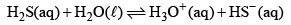K = 5 × 10–19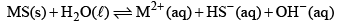Detailed Solution for Chemistry - 2013 Past Year Paper - Question 23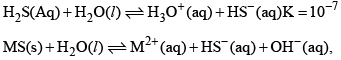K = 5 × 10–19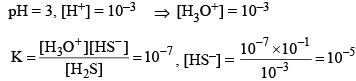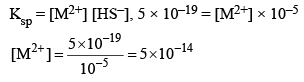Therefore, solubility of metal sulphide = 5 × 10–14 M.

Chemistry - 2013 Past Year Paper - Question 24

For each of the following metallo-proteins identify the metal-ion at the active-site and the function of the proteins: deoxy-hemoglobin, deoxy-myoglobin, oxy-hemocyanin, cytochrome-C and carbonic anhydrase.

Detailed Solution for Chemistry - 2013 Past Year Paper - Question 24

Deoxy-hemoglobin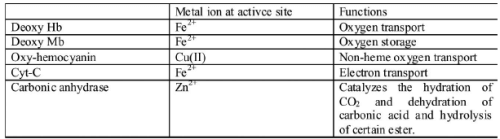Chemistry - 2013 Past Year Paper - Question 25

A solution containing 250 ppm of CuSO4 · 5H2O (formula weight = 250) has an absorbance of 0.1 measured in 1 cm cell at 600 nm. Calculate the molar absorptivity (e) of CuSO4 · 5H2O in L M-1 cm-1 . When 25 mL of Na2EDTA (aq) solution is titrated against Na2EDTA (aq) solution, it consumes at 50 mL of Na2EDTA (aq) solution. Calculate the concentration of Na2EDTA (aq) solution in moles L-1 .

Detailed Solution for Chemistry - 2013 Past Year Paper - Question 25

C = 250 ppm = 10–3 mol ltr.
A = 0.1, x = 1 cm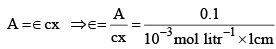= 102 litr mol-1 cm -1

Chemistry - 2013 Past Year Paper - Question 26

Assume the complex [NI(PPh3)2(SCN)2] is paramagnetic. The analogous complex of Pd(II) is diamagnetic. Draw all the probable isomers for both the complexes considering SCN is an ambidentate ligand.

Detailed Solution for Chemistry - 2013 Past Year Paper - Question 26

[Ni(PPh3)2(SCN)] → Paramagnetic, therefore, tetrahedral Isomers: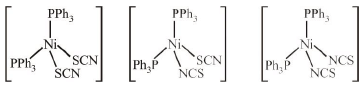[Pd(PPh3)2(SCN)2] → Diamagnetic, therefore, square planar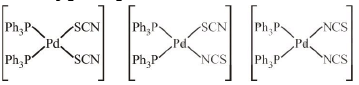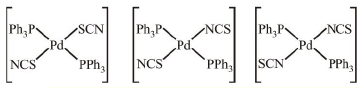Chemistry - 2013 Past Year Paper - Question 27

Write the structure of A to E in the following reaction sequence: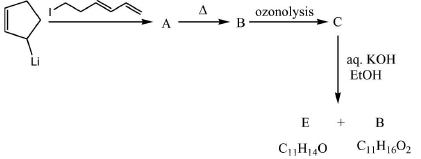Detailed Solution for Chemistry - 2013 Past Year Paper - Question 27

Chemical transformation involved in above chemical reaction can be illustrated as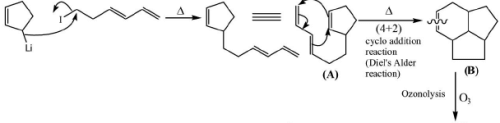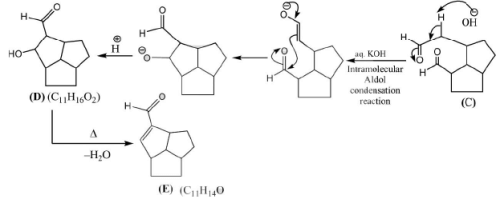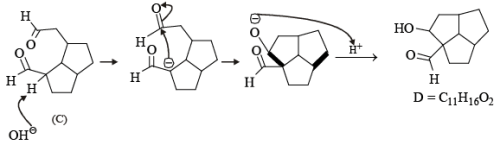Chemistry - 2013 Past Year Paper - Question 28

Write the structures of F to J in the following reaction scheme: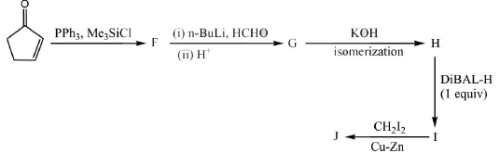[DiBAL-H = diisobutylaluminium hydride]

Detailed Solution for Chemistry - 2013 Past Year Paper - Question 28

Chemical transformation involved in above chemical reaction can be illustrated as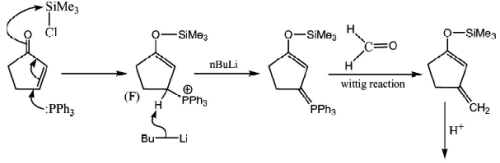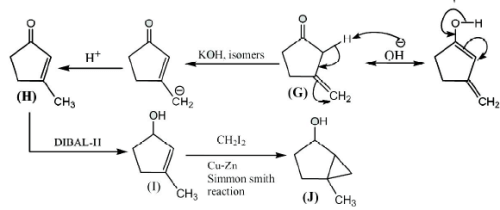Chemistry - 2013 Past Year Paper - Question 29

Propose a mechanism for the following reaction. Show stepwise correct reactive intermediates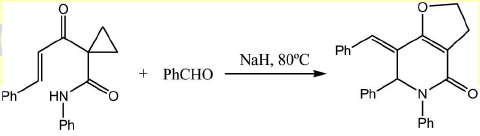Detailed Solution for Chemistry - 2013 Past Year Paper - Question 29

Chemical transformation involved in above chemical reaction can be illustrated as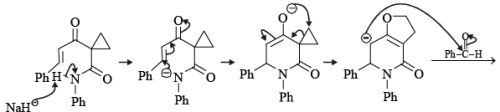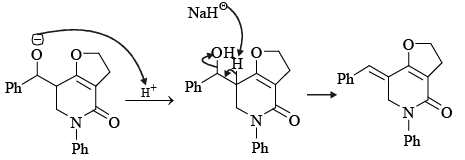Chemistry - 2013 Past Year Paper - Question 30

Complete the following reaction sequence and write structures of K to O.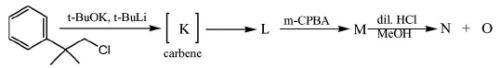Detailed Solution for Chemistry - 2013 Past Year Paper - Question 30

Chemical transformation involved in above chemical reaction can be illustrated as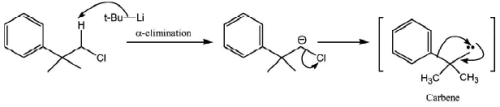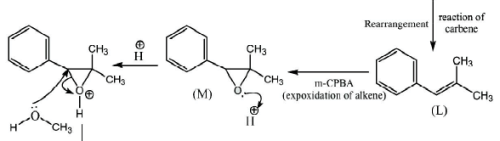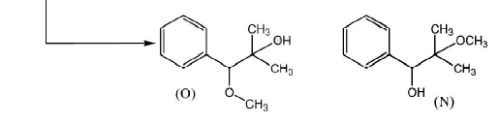## IIT JAM Past Year Papers and Model Test Paper (All Branches)

8 docs|48 tests
Information about Chemistry - 2013 Past Year Paper Page
In this test you can find the Exam questions for Chemistry - 2013 Past Year Paper solved & explained in the simplest way possible. Besides giving Questions and answers for Chemistry - 2013 Past Year Paper, EduRev gives you an ample number of Online tests for practice

## IIT JAM Past Year Papers and Model Test Paper (All Branches)

8 docs|48 tests(Scan QR code)